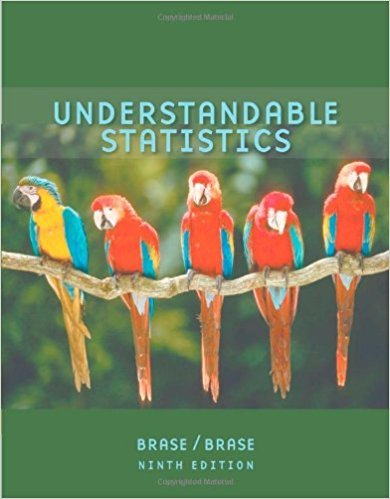×
×

# Solutions for Chapter 2.2: Organizing Data## Full solutions for Understandable Statistics | 9th Edition

ISBN: 9780618949922Solutions for Chapter 2.2: Organizing Data

Solutions for Chapter 2.2
4 5 0 298 Reviews
17
5
##### ISBN: 9780618949922

This textbook survival guide was created for the textbook: Understandable Statistics, edition: 9. Understandable Statistics was written by and is associated to the ISBN: 9780618949922. Since 12 problems in chapter 2.2: Organizing Data have been answered, more than 36168 students have viewed full step-by-step solutions from this chapter. Chapter 2.2: Organizing Data includes 12 full step-by-step solutions. This expansive textbook survival guide covers the following chapters and their solutions.

Key Statistics Terms and definitions covered in this textbook
• 2 k p - factorial experiment

A fractional factorial experiment with k factors tested in a 2 ? p fraction with all factors tested at only two levels (settings) each

A variation of the R 2 statistic that compensates for the number of parameters in a regression model. Essentially, the adjustment is a penalty for increasing the number of parameters in the model. Alias. In a fractional factorial experiment when certain factor effects cannot be estimated uniquely, they are said to be aliased.

• Arithmetic mean

The arithmetic mean of a set of numbers x1 , x2 ,…, xn is their sum divided by the number of observations, or ( / )1 1 n xi t n ? = . The arithmetic mean is usually denoted by x , and is often called the average

• Average run length, or ARL

The average number of samples taken in a process monitoring or inspection scheme until the scheme signals that the process is operating at a level different from the level in which it began.

• Box plot (or box and whisker plot)

A graphical display of data in which the box contains the middle 50% of the data (the interquartile range) with the median dividing it, and the whiskers extend to the smallest and largest values (or some deined lower and upper limits).

• Chance cause

The portion of the variability in a set of observations that is due to only random forces and which cannot be traced to speciic sources, such as operators, materials, or equipment. Also called a common cause.

• Correction factor

A term used for the quantity ( / )( ) 1 1 2 n xi i n ? = that is subtracted from xi i n 2 ? =1 to give the corrected sum of squares deined as (/ ) ( ) 1 1 2 n xx i x i n ? = i ? . The correction factor can also be written as nx 2 .

• Correlation matrix

A square matrix that contains the correlations among a set of random variables, say, XX X 1 2 k , ,…, . The main diagonal elements of the matrix are unity and the off-diagonal elements rij are the correlations between Xi and Xj .

• Crossed factors

Another name for factors that are arranged in a factorial experiment.

• Defect

Used in statistical quality control, a defect is a particular type of nonconformance to speciications or requirements. Sometimes defects are classiied into types, such as appearance defects and functional defects.

• Degrees of freedom.

The number of independent comparisons that can be made among the elements of a sample. The term is analogous to the number of degrees of freedom for an object in a dynamic system, which is the number of independent coordinates required to determine the motion of the object.

• Density function

Another name for a probability density function

• Discrete random variable

A random variable with a inite (or countably ininite) range.

• Discrete uniform random variable

A discrete random variable with a inite range and constant probability mass function.

• Erlang random variable

A continuous random variable that is the sum of a ixed number of independent, exponential random variables.

• Experiment

A series of tests in which changes are made to the system under study

• F distribution.

The distribution of the random variable deined as the ratio of two independent chi-square random variables, each divided by its number of degrees of freedom.

• False alarm

A signal from a control chart when no assignable causes are present

• Fisher’s least signiicant difference (LSD) method

A series of pair-wise hypothesis tests of treatment means in an experiment to determine which means differ.

• Goodness of fit

In general, the agreement of a set of observed values and a set of theoretical values that depend on some hypothesis. The term is often used in itting a theoretical distribution to a set of observations.

×